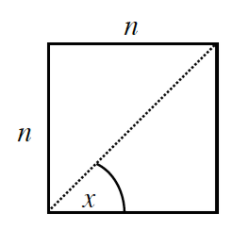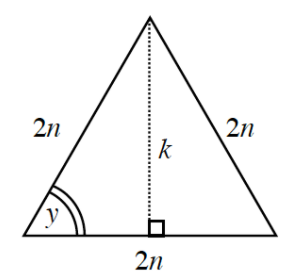### Home > PC3 > Chapter 1 > Lesson 1.3.1 > Problem1-117

1-117.

Use the Pythagorean Theorem and the diagrams below to answer the following questions.1. The square at right has sides of length $n$. What is the length of the diagonal in terms of $n$? Use exact values.

Use the Pythagorean Theorem.
$n² + n² = d²$
Solve for $d$.

2. What is the measure of the angle labeled $x$?

What do you know about the interior angles of squares?

3. The triangle at right is equilateral with each with sides of length $2n$. What is the height $(k)$ in terms of $n$? Use exact values.

The base is made into two equal parts by $k$
Use the Pythagorean Theorem to write an equation and solve for k.

4. What is the measure of the angle labeled $y$?

What do you know about the interior angles of an equilateral triangle?